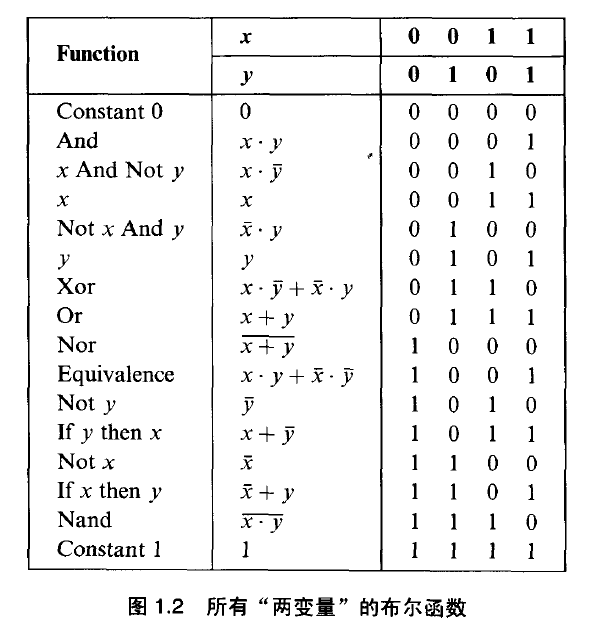## HCL和布尔表达式

HCL类似于用布尔表达式来书写门电路的组合方式, 像这个`bool eq = (a && b) || (!a && !b);`. 离散数学中提到, 不管多复杂的逻辑电路, 只要有了and or not三个算子, 就是完备的, 可以拼接出来.

### 练习 4.9 写出xor的HCL表达式```bool xor = (a && !b) || (!a && b)

```

HCL语言看上去很像C语言表达式, 相比C语言, HCL有一些特点:

1. HCL的值是持续响应的, 并不是像语句一样遇到才求值
2. C的判断条件是不是0都是真, 而是0就是假. HCL中的值只有0和1代表高低电压, 不存在其他值.
3. C表达式有短路作用, 而HCL一直响应输入变化, 不存在求值与否的问题.
4. 将所有输入都假设为数据类型是int的字来解释, 其实底层是没有数据类型一说的, 这样假设是为了说起来方便.
5. 允许比较字是否相等, 即 bool eq = (A==B)

## 多路复用器

```[
select1 : expr1;
select2 : expr2;
select3 : expr3;
.
.
.
selectN : exprN;
]
```

selectN是选择表达式, 即这个表达式如果为1, 就被选中, 之后的输出结果就是对应的exprN. 注意, 逻辑电路在同一时刻必定有一个输出, 所以MUX2一般会写成:

```word out = [
s: A;
1: B;
]
```

```word out4 = [
!s1 && !s0 : A;  00的情况
!s1        : B;  01的情况, 由于第一种情况要求!s1和!s0都为真, 既然不满足条件, 说明两个里边至多一个为真, 因此这里无需判断!s0
!s0        : C;  10的情况, 和上一行一样, 无需判断!s1
1          : D;  11, 默认情况, 也就是!s1和!s0都是0的情况.
]
```

### 练习 4.11 逻辑游戏

```word min3 = [
A <= B && A <= C : A;
B <= C           : B;
1                : C;
]
```

### 练习 4.12 找中间值

```word middle3 = [
A <= B && B <= C : B;
C <= B && B <= A : B;
B <= A && A <= C : A;
C <= A && A <= B : A;
1: C;  前两个情况都不满足,就是C了
]
```

## 集合

```bool s1 = code == 2 || code == 3
bool s0 = code == 1 || code == 3
```

```bool s1 = code in {2, 3}
bool s0 = code in {1, 3}
```